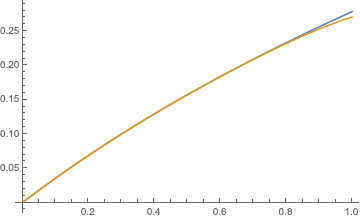# Lambert W function multiplied by a constant

Consider the equation

$$y=x e^x.$$ Its real solution is given by $$x=W(y),$$ where $$W$$ is the Lambert W function (or product log).

Can the function

$$f(x) = W\left(-\frac{1}{r}xe^x\right)$$

be written in simple terms of $$x$$?

What I've tries so for is writing this as a power series expansion

$$W(x)=\sum_{n=1}^{\infty}\frac{(-1)^n n^{n-2}}{(n-1)!}x^n$$

therefore with the constant I've introduced $$\begin{split} W\left(-\frac{1}{r}x\right) & =\sum_{n=1}^{\infty}\frac{(-1)^{n-1} n^{n-2}}{(n-1)!}\left(-\frac{x}{r}\right)^n\\ & =\sum_{n=1}^{\infty}-\frac{(-1)^{n-1}(-1)^{n-1} n^{n-2}}{(n-1)!}\left(\frac{x}{r}\right)^n \\ & =\sum_{n=1}^{\infty}-\frac{n^{n-2}}{(n-1)!}\left(\frac{x}{r}\right)^n \end{split}$$ but got stuck here.

I've also tried guessing many functions in mathematica that yeilded no result.

As I see it now the problem is not solvable

• One thing to be aware of is that the domain of the Lambert function isn't closed under $y \mapsto -\frac1 r y$, so you'll have to be careful about where your $f$ is defined. – LSpice Jul 1 '19 at 20:25
• @LSpice, $x$ is positive and real, and $r>0$, does that help? – jarhead Jul 1 '19 at 20:27
• Probably not much; still the function is defined only on $(0, W(r/e))$. – LSpice Jul 1 '19 at 20:28
• What have you tried? Did you graph the function? Did you calculate a few terms of its Taylor series? If so, what did you see? – Daniel McLaury Jul 1 '19 at 21:34
• I did not downvote the post. – Daniel McLaury Jul 2 '19 at 4:46

## 3 Answers

If what you want is a series expansion in powers of $$x$$ and $$t:=1/r$$, we can write $$f(x,t)=W\big(- {txe^x}\big)=-\sum_{n=1}^\infty {q_n(t)} \,x^n=-\bigg[tx+\frac{2t+2t^2}{2!}\, {x^2} +\frac{3t+12t^2+9t^3}{3!}\,x^3+\dots\bigg]$$ The coefficient $$q_n$$ is a polynomial of degree $$n$$, precisely $$q_n(t)=\frac{1}{n!}(tD)^{n-1}(1+t)^n$$ where $$D$$ denotes differentiation in $$t$$.

• I did not understand what you have done, $r$ is a parameter. I'm also interested in a closed form, or the closest thing I can get to it. Getting to a closed form through a power series is one option out of many I was hoping people would lay out here. – jarhead Jul 9 '19 at 15:10
• I just wrote the power series expansion of $f(x)=W(-x e^x/r)=\sum_{n=1}^\infty q_nx^n$. Of course he coefficients $q_n$ depend on the parameter $r$; in fact they are polynomials in $t:=1/r$. – Pietro Majer Jul 9 '19 at 15:15
• @jarhead -- the closed form exists, it is $y = W(-r^{-1}xe^x)$, resumming the power series, either this one or the one in my answer, will just give you back that closed-form expression. – Carlo Beenakker Jul 9 '19 at 15:16
• Indeed it seems difficult to have a better closed form. Instead, I would look at the dependence from $r$; for instance, if I'm not wrong, $f_s\circ f_r=f_r\circ f_s=W\circ f_{-sr}$ – Pietro Majer Jul 9 '19 at 15:39
• Can you please elaborate on what is $D$, I apologize as it seems basic, but if I take a derivative of $r$ with respect to $x$ then I will get $0$ as it is a parameter. – jarhead Jul 10 '19 at 7:52

Maple did the following. (Of course once you see it you can do it yourself...)

$$y = W(-\frac{1}{r}xe^x)$$ solve for $$x$$; result $$x = W(-rye^y)$$

Probably even your original assertion needs adjustment. We may think that $$W(xe^x) = x$$ but perhaps not. Here is the graph for $$W_0(xe^x)$$Only part of that is $$y=x$$.

For more explanation, see this, where $$W_0(xe^x)$$ is in red and $$W_{-1}(xe^x)$$ is in blue:• thanks for your effort but this not address the question. – jarhead Jul 2 '19 at 12:23
• also I'm only worried about $x>0$ so the $-1$ real branch is not an issue. Still no solution. – jarhead Jul 2 '19 at 12:33
• I expect the best solution is $x = W(-rye^y)$ which has no simpler form for general $r$. – Gerald Edgar Jul 2 '19 at 13:15

As mentioned by Gerald Edgar, you seek to simplify $$y = W(-r^{-1}xe^x)\Rightarrow x=W(-rye^y),$$ for $$r>0$$. Here is a series expansion in powers of $$r$$,

$$x=-\sum_{n=1}^\infty n^{n-1}\frac{1}{n!}(rye^y)^n.$$

The plot compares the exact result for $$x$$ (blue curve) with the series expansion up to $$n=8$$ (gold) at $$y=-1$$, as a function of $$r$$. (I take $$y<0$$ because the OP says the interest is in $$x>0$$.) The series expansion remains quite accurate even for $$r$$ approaching unity.• and can you transform this series to obtain a continuous function of $f(x)$ when $y=f(x)$? – jarhead Jul 4 '19 at 16:54
• I'm not quite sure what you mean; the series $\sum_{n=1}^\infty n^{n-1}\frac{1}{n!}(rye^y)^n$ is a contiuous function of $y$; if you are asking whether the series can be summed in closed form, the answer is "yes", but that would bring you back to the Lambert W-function, which you wish to avoid. – Carlo Beenakker Jul 4 '19 at 17:46
• please refer again to my question – jarhead Jul 5 '19 at 9:09